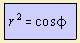# dipole curve

## sexticGiven two electrostatic equal but opposite charges which are close to each other (an electrostatic dipole). The equipotential is then formed by the dipole curve 1).

The equation in Cartesian coordinates can be written as (x2 + y2)3 = x2.

notes

1) Let charges be at A and B on the x-axis, and P being the point for which the potential is calculated.

Then the equation of the equipotential starts with 1/PA - 1/PB = constant.
Now 1/PA - 1/PB = PB2 - PA2 / PA PB (PA + PB), which is proportional toThe last expression is equal to AB . cosφ/r2, which had to be proved.